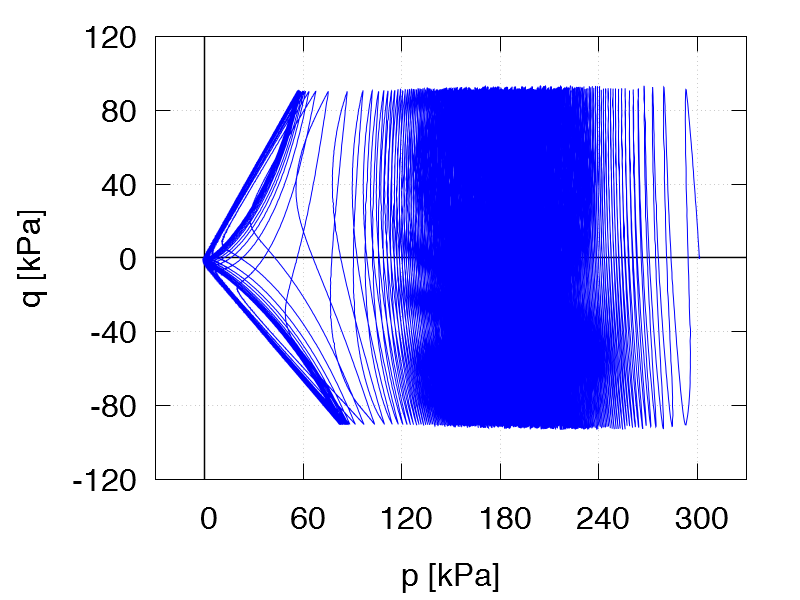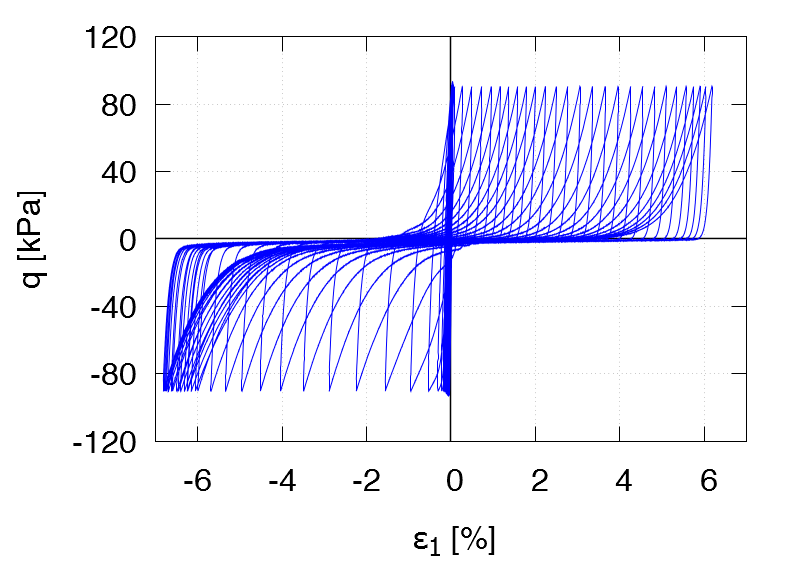Undrained cyclic tests with isotropic consolidation and stress cycles:   Data of all tests of this series
 TCUI1 ID0 = 0.27 p0 = 200 kPa qampl = 30 kPa Test data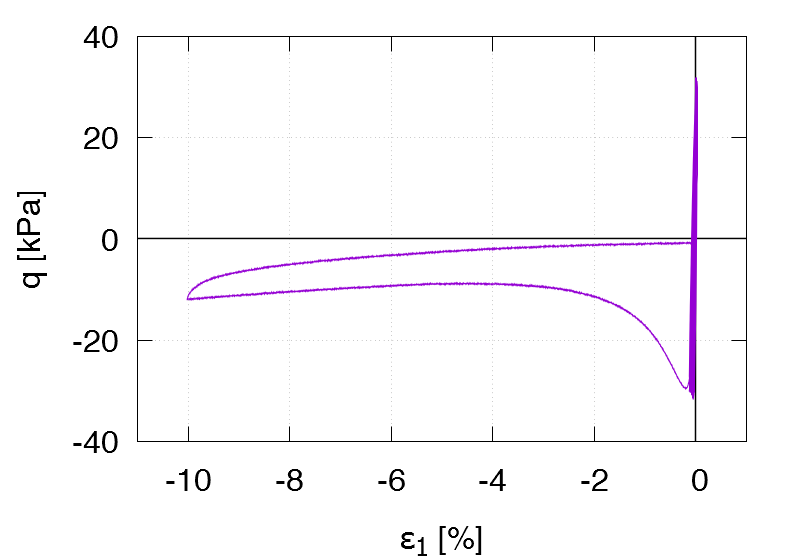TCUI2 ID0 = 0.25 p0 = 100 kPa qampl = 15 kPa 1st drained cycle Test data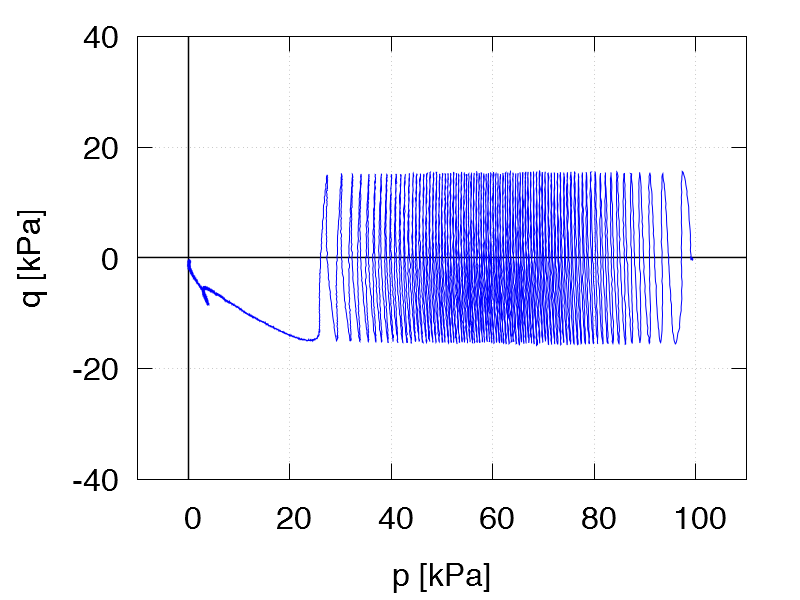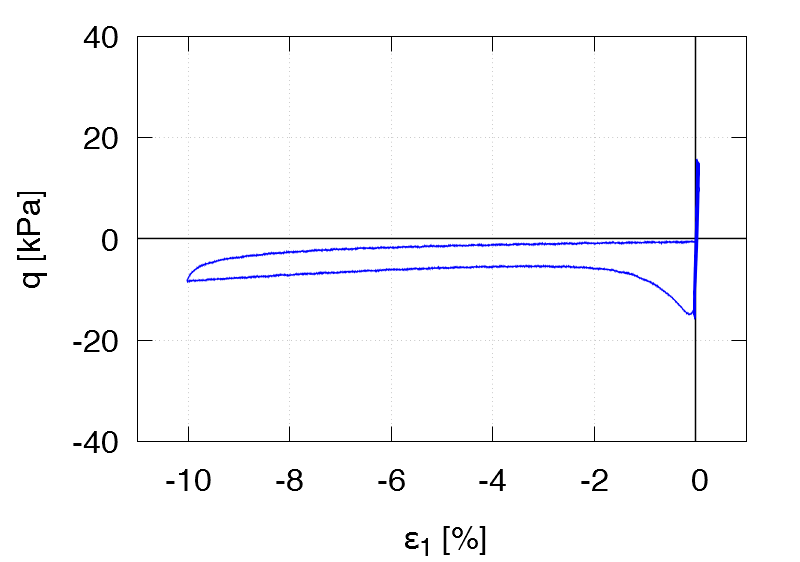TCUI3 ID0 = 0.28 p0 = 100 kPa qampl = 20 kPa 1st drained cycle Test data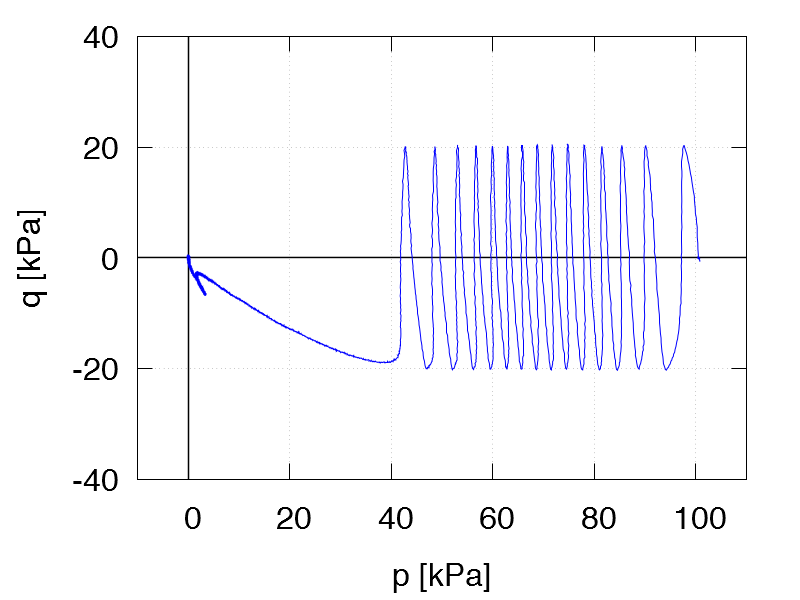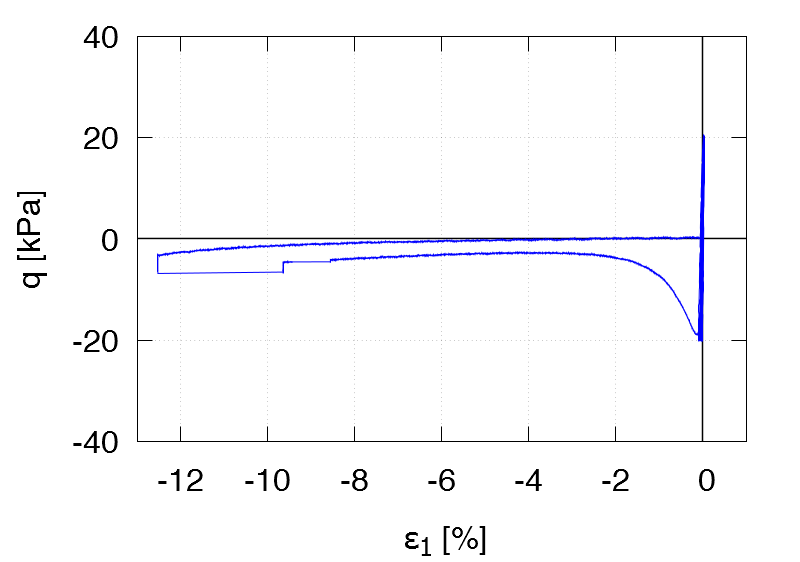TCUI4 ID0 = 0.25 p0 = 100 kPa qampl = 25 kPa 1st drained cycle Test data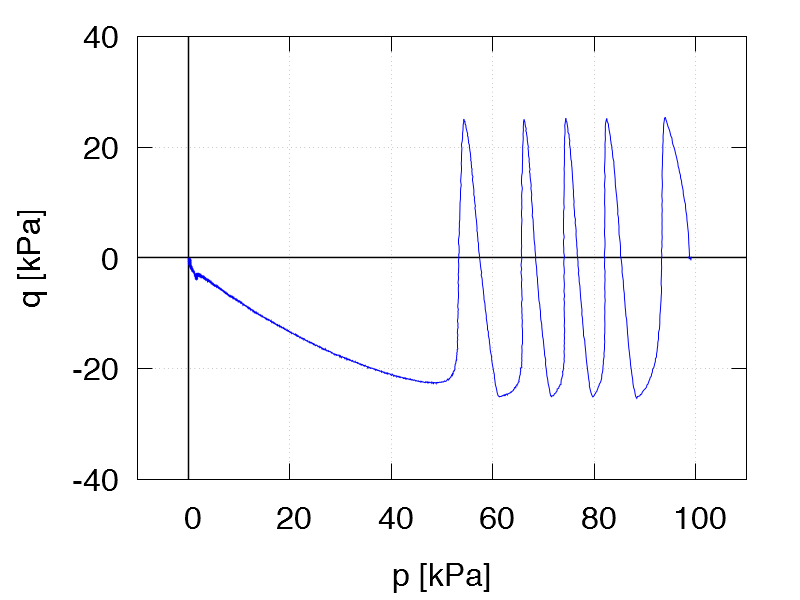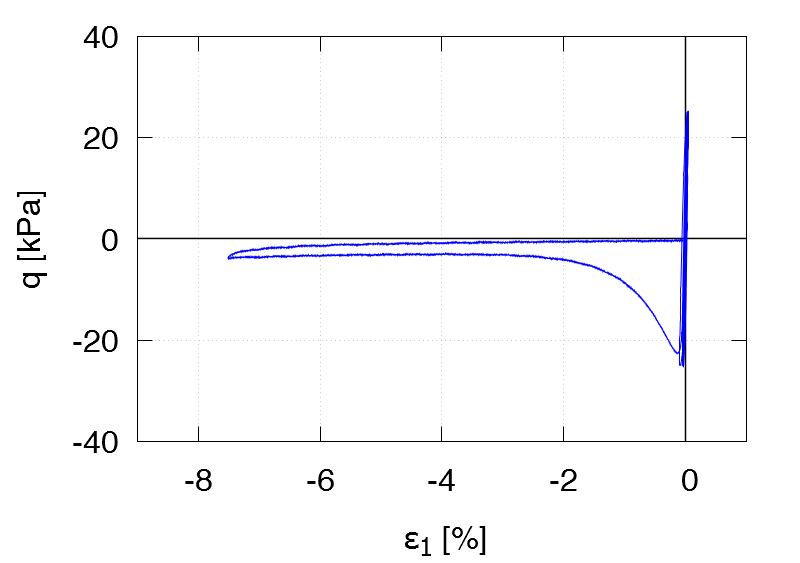TCUI5 ID0 = 0.26 p0 = 200 kPa qampl = 30 kPa 1st drained cycle Test data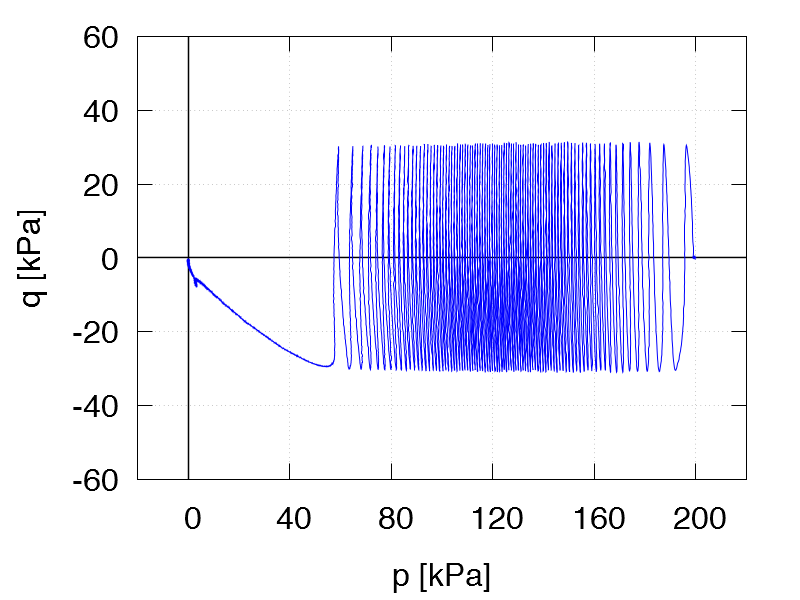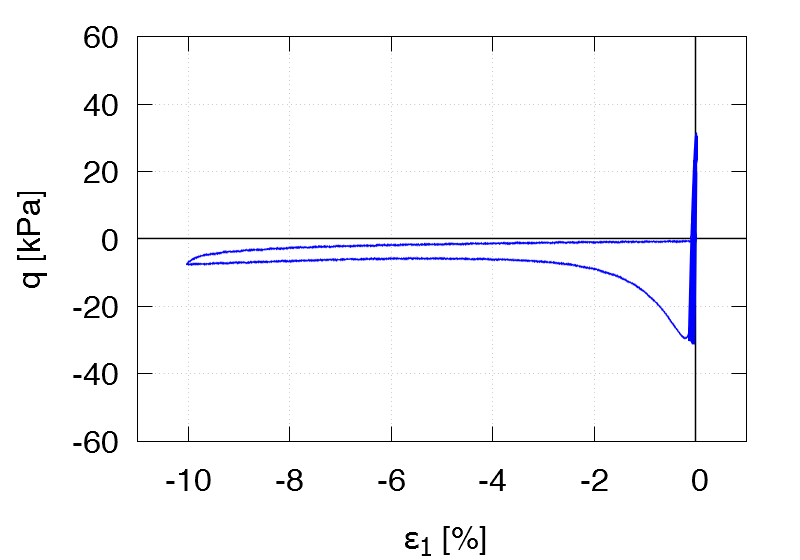TCUI6 ID0 = 0.24 p0 = 300 kPa qampl = 45 kPa 1st drained cycle Test data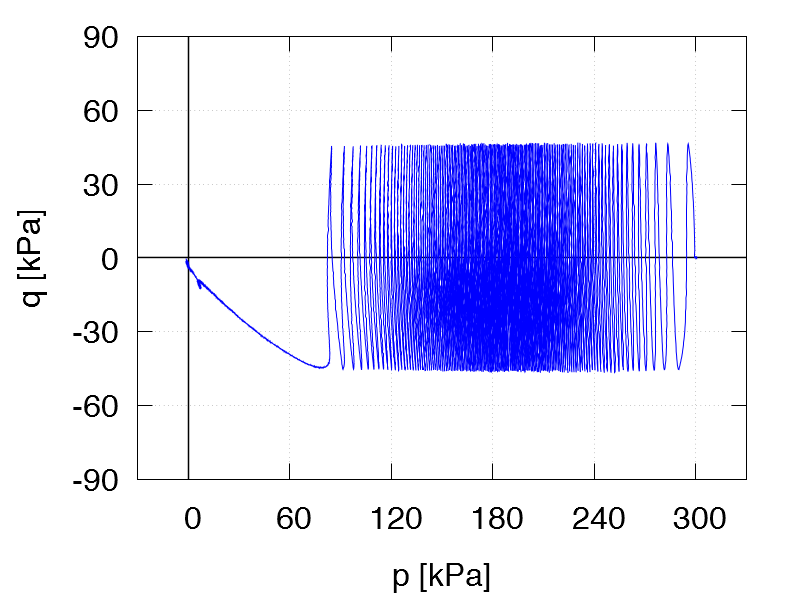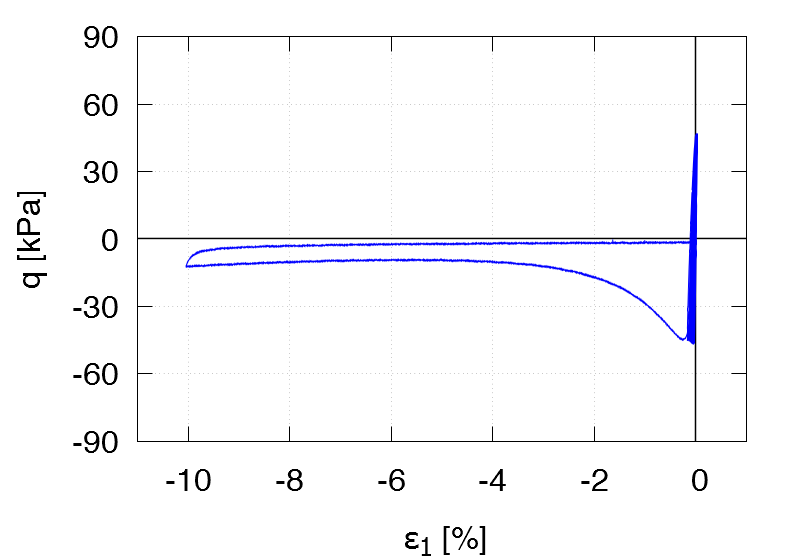TCUI7 ID0 = 0.67 p0 = 200 kPa qampl = 60 kPa Test data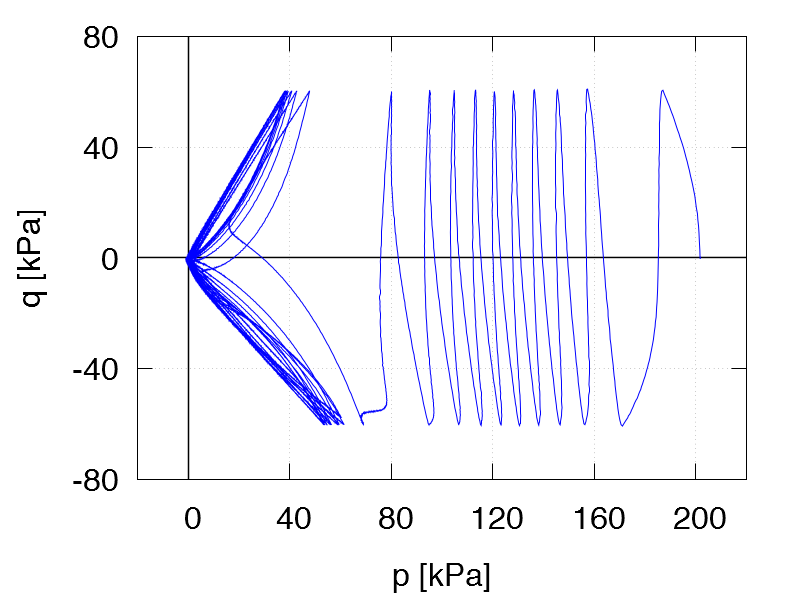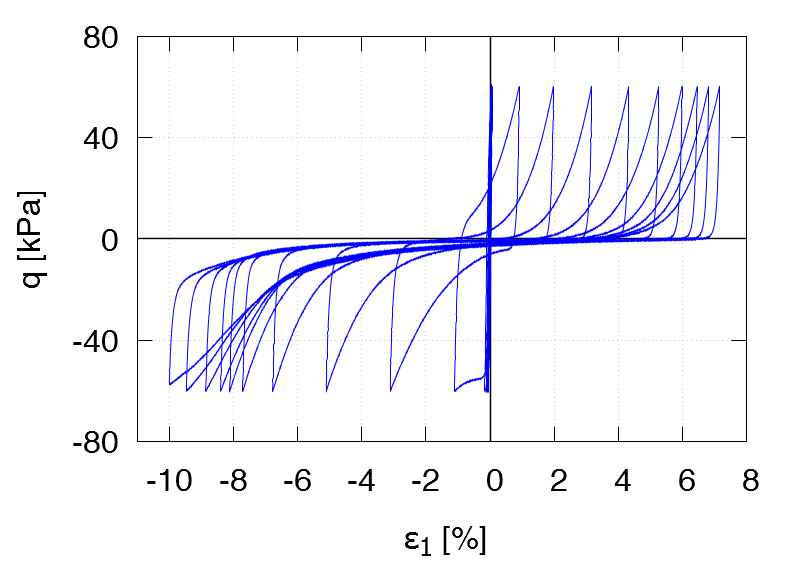TCUI8 ID0 = 0.62 p0 = 100 kPa qampl = 20 kPa 1st drained cycle Test data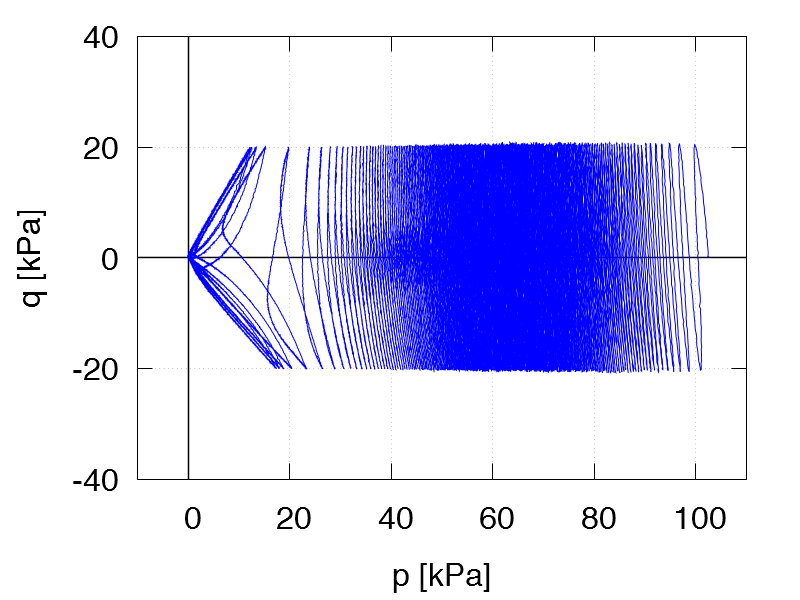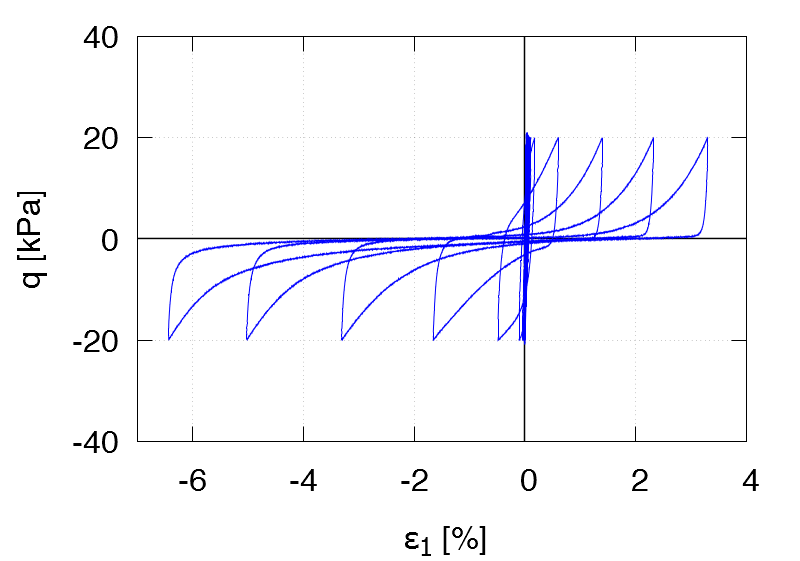TCUI9 ID0 = 0.68 p0 = 100 kPa qampl = 25 kPa 1st drained cycle Test data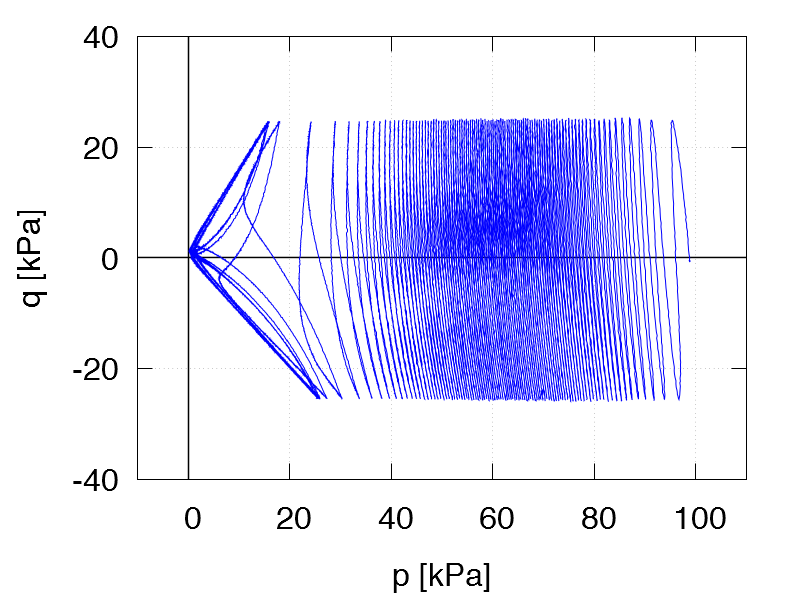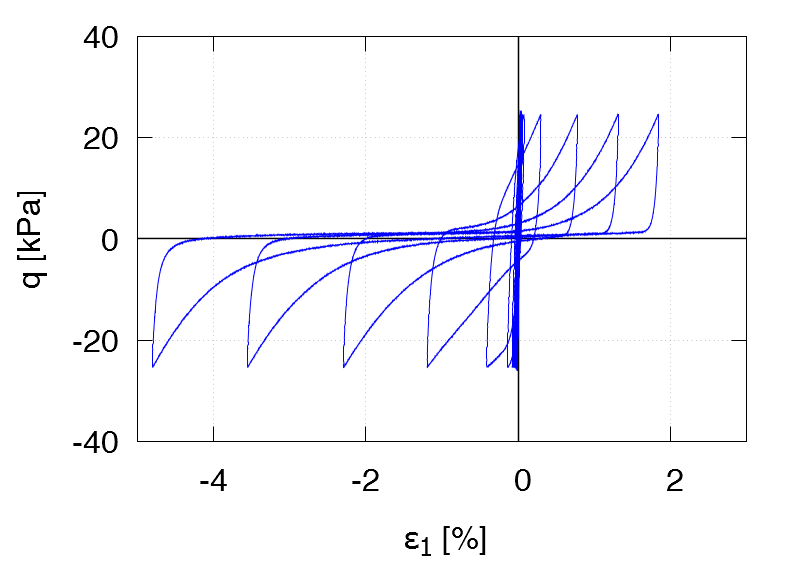TCUI10 ID0 = 0.61 p0 = 100 kPa qampl = 30 kPa 1st drained cycle Test data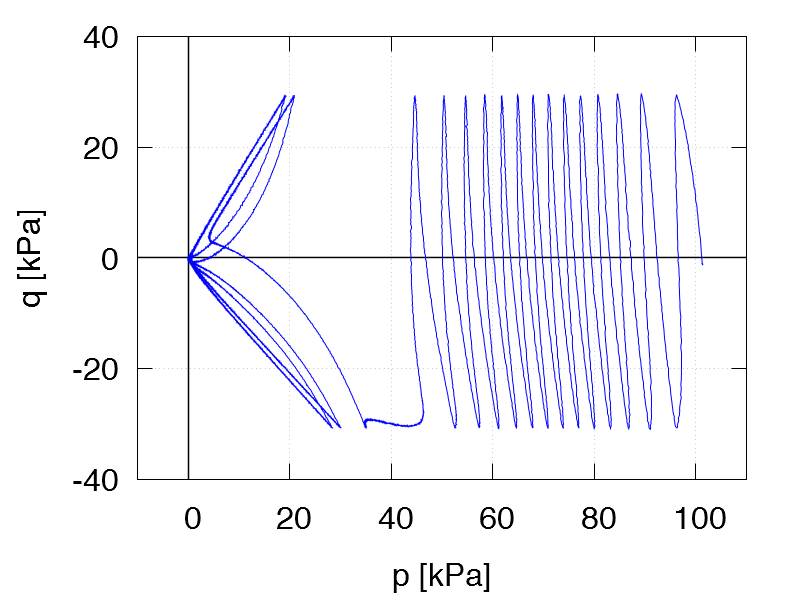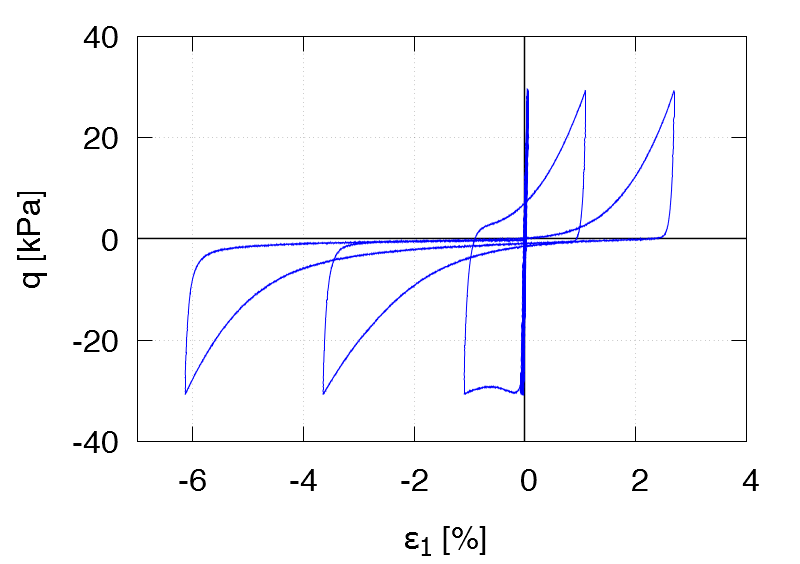TCUI11 ID0 = 0.56 p0 = 200 kPa qampl = 40 kPa 1st drained cycle Test data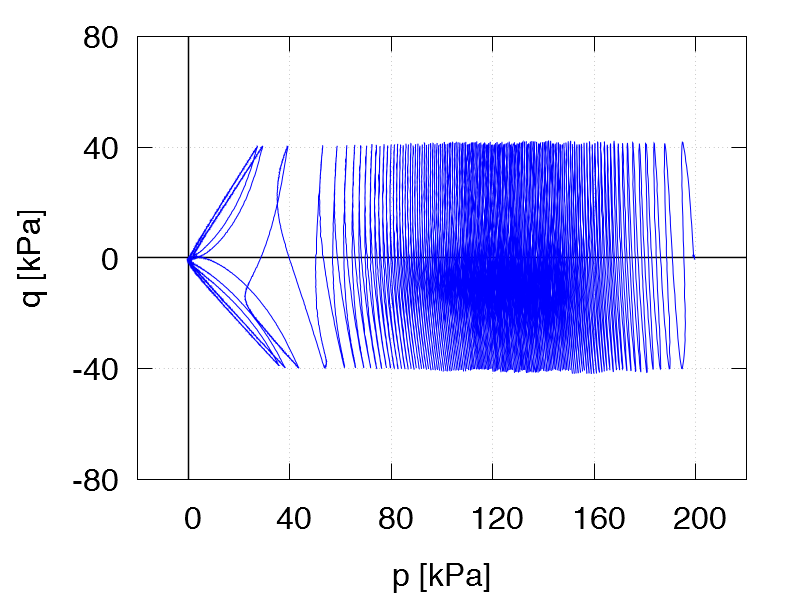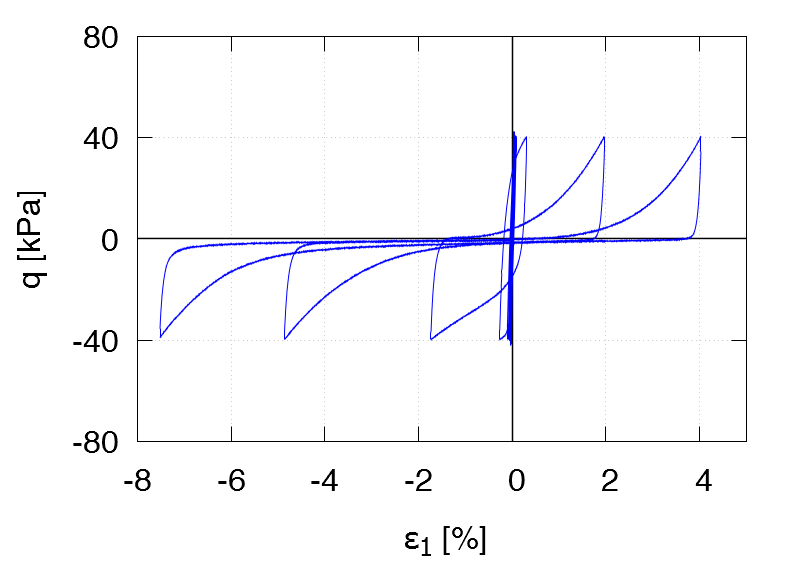TCUI12 ID0 = 0.64 p0 = 200 kPa qampl = 50 kPa 1st drained cycle Test data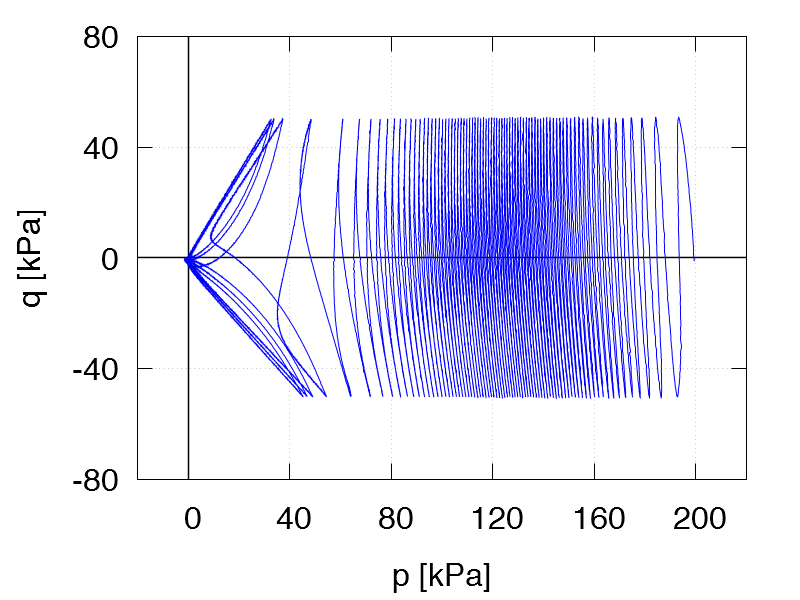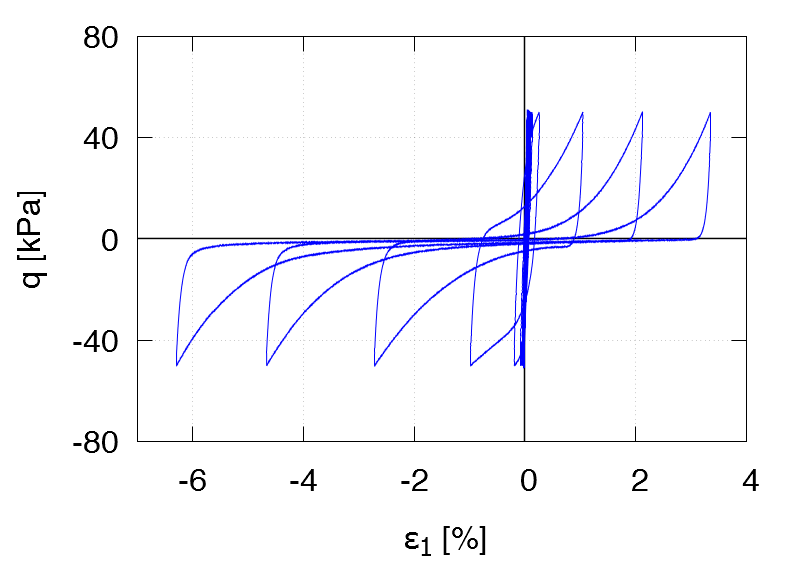TCUI13 ID0 = 0.59 p0 = 200 kPa qampl = 60 kPa 1st drained cycle Test data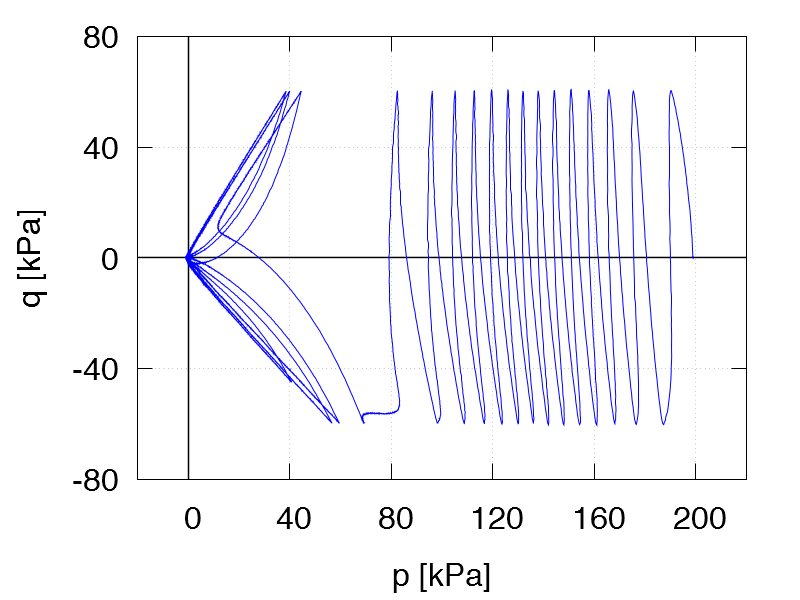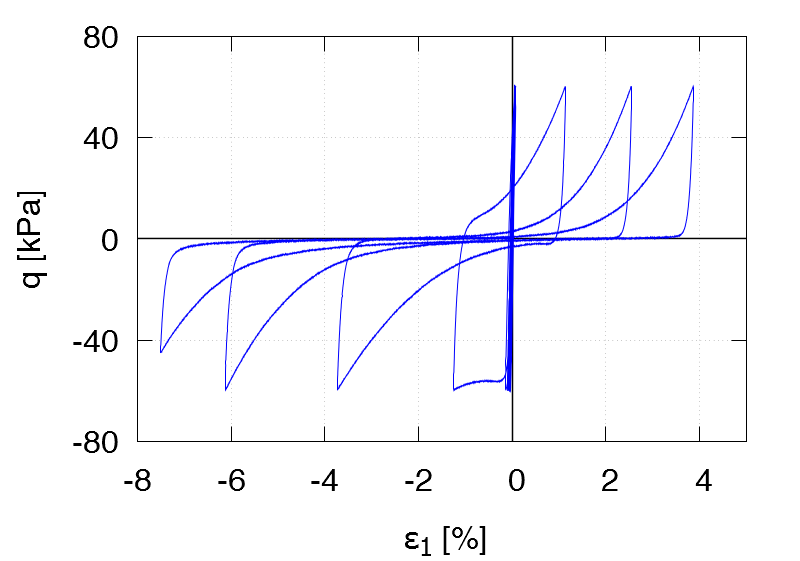TCUI14 ID0 = 0.55 p0 = 300 kPa qampl = 60 kPa 1st drained cycle Test data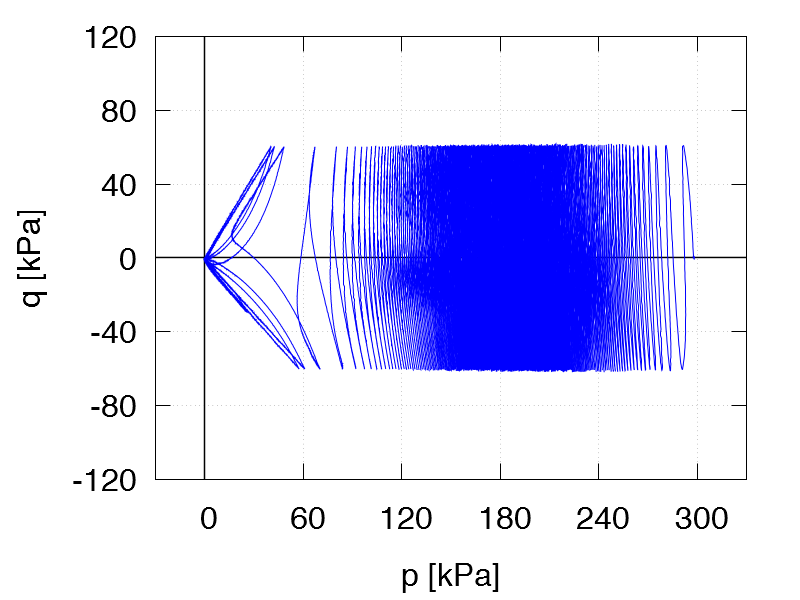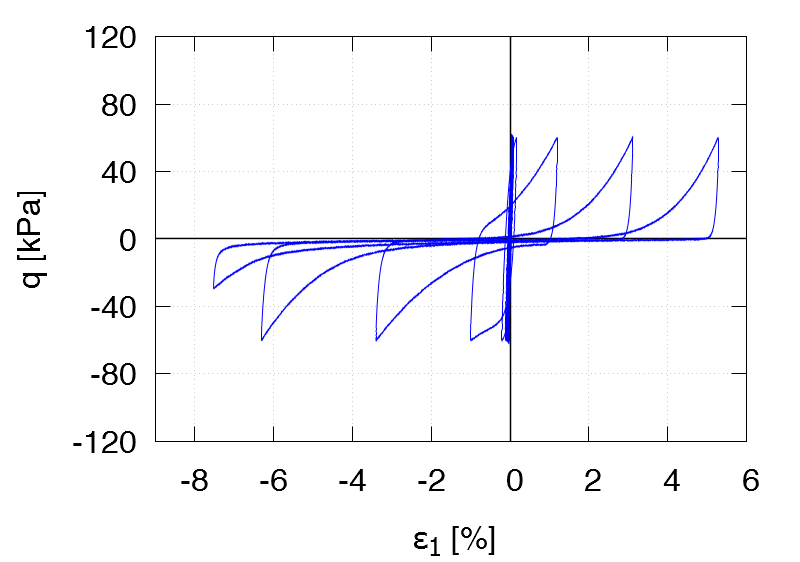TCUI15 ID0 = 0.65 p0 = 300 kPa qampl = 75 kPa 1st drained cycle Test data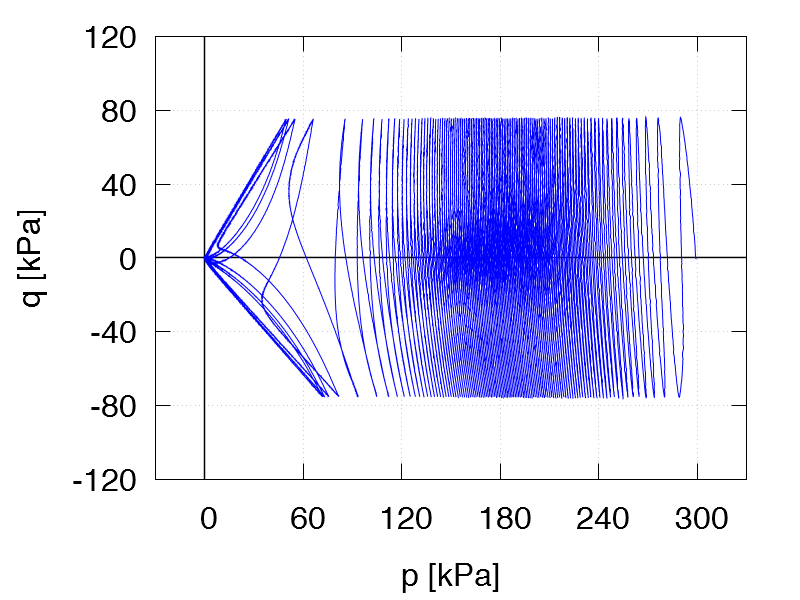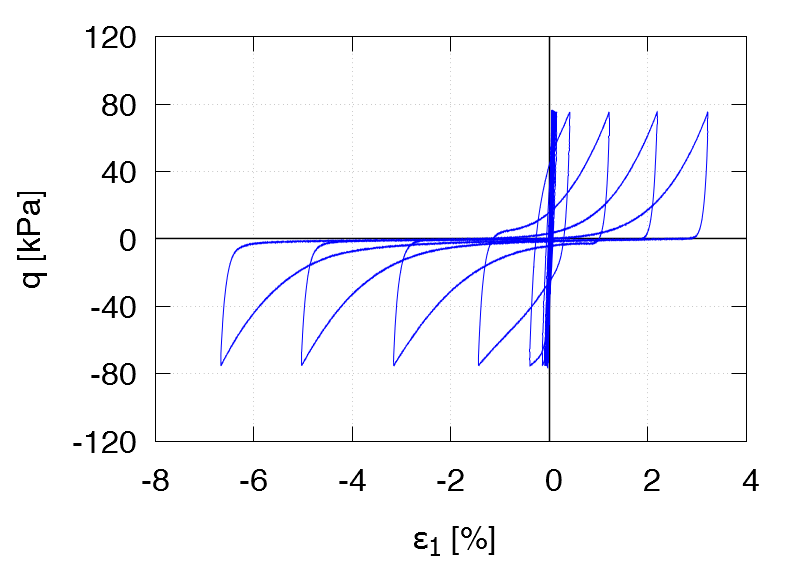TCUI16 ID0 = 0.63 p0 = 300 kPa qampl = 90 kPa 1st drained cycle Test data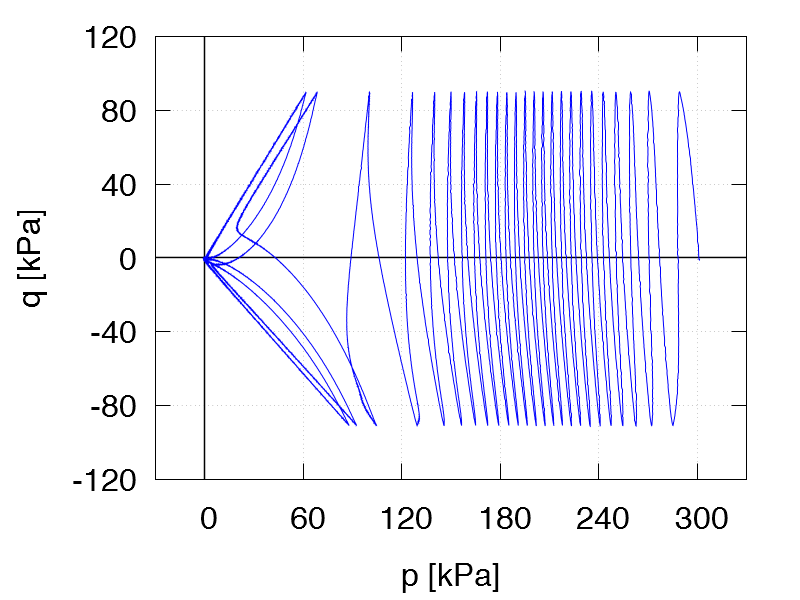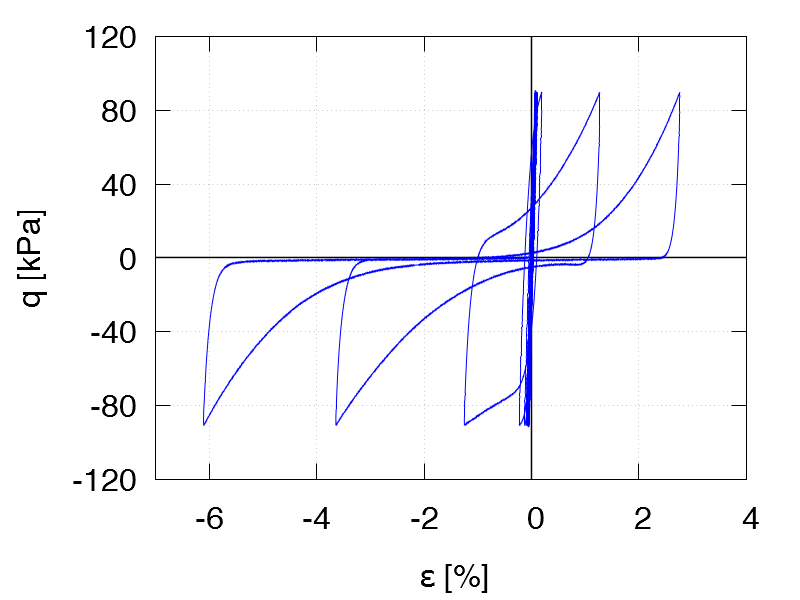TCUI17 ID0 = 0.87 p0 = 200 kPa qampl = 60 kPa Test data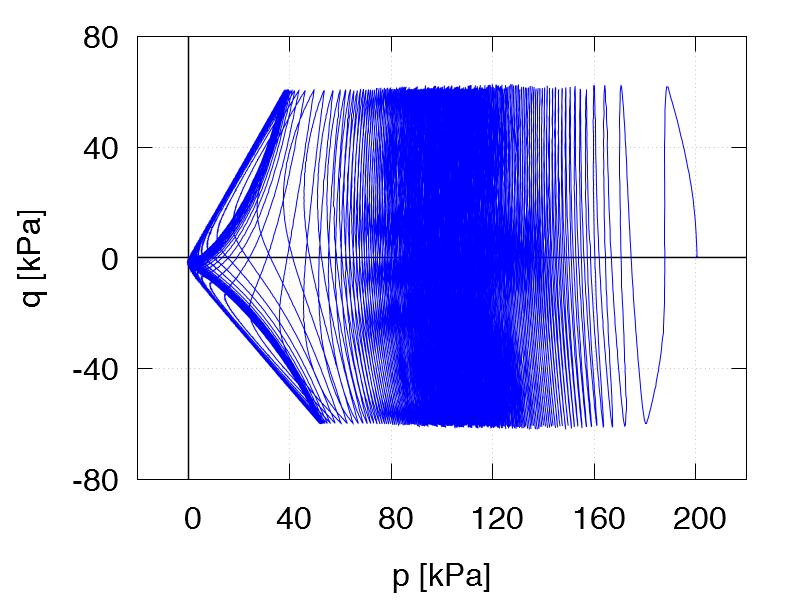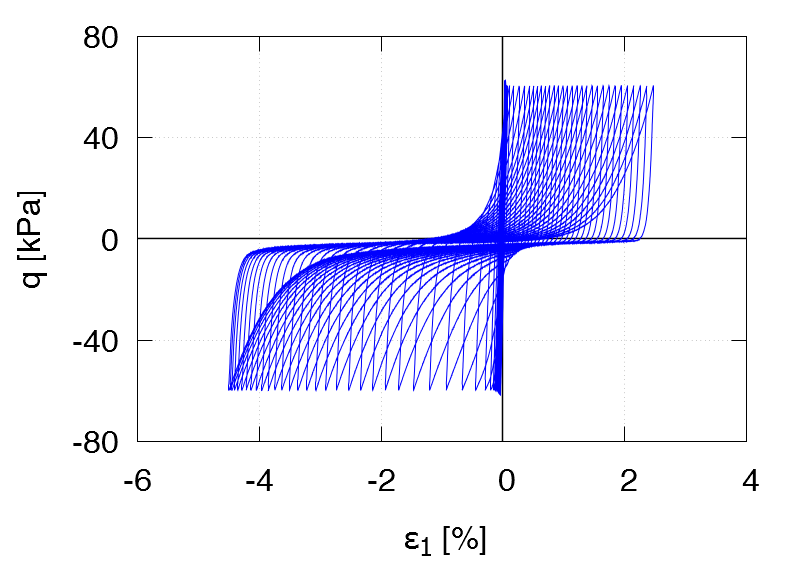TCUI18 ID0 = 0.78 p0 = 100 kPa qampl = 30 kPa 1st drained cycle Test data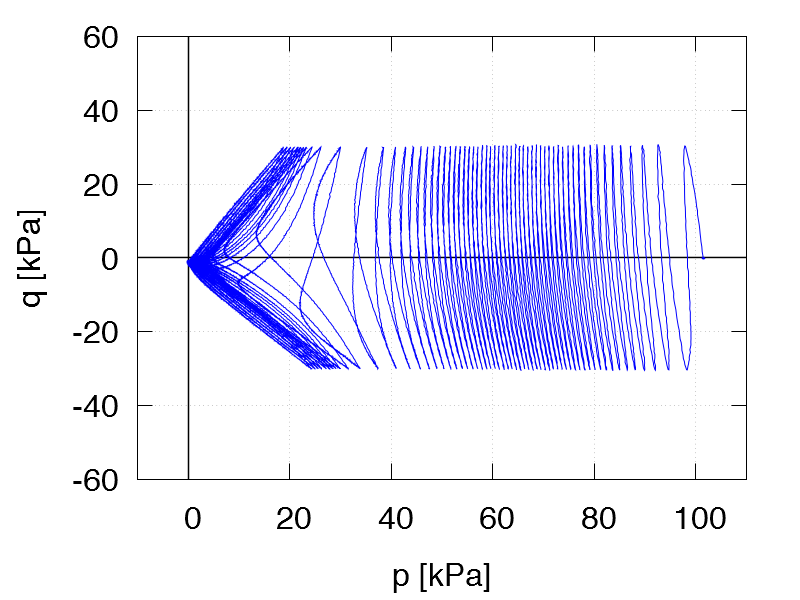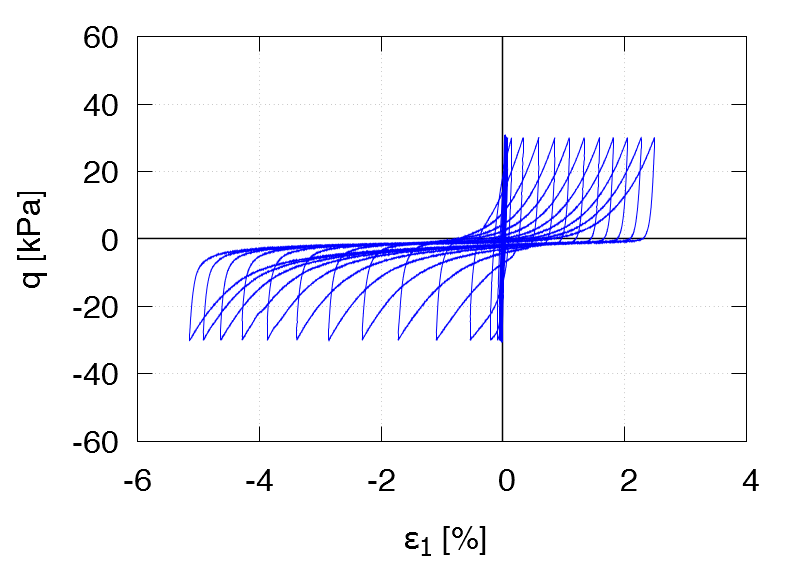TCUI19 ID0 = 0.78 p0 = 100 kPa qampl = 40 kPa 1st drained cycle Test data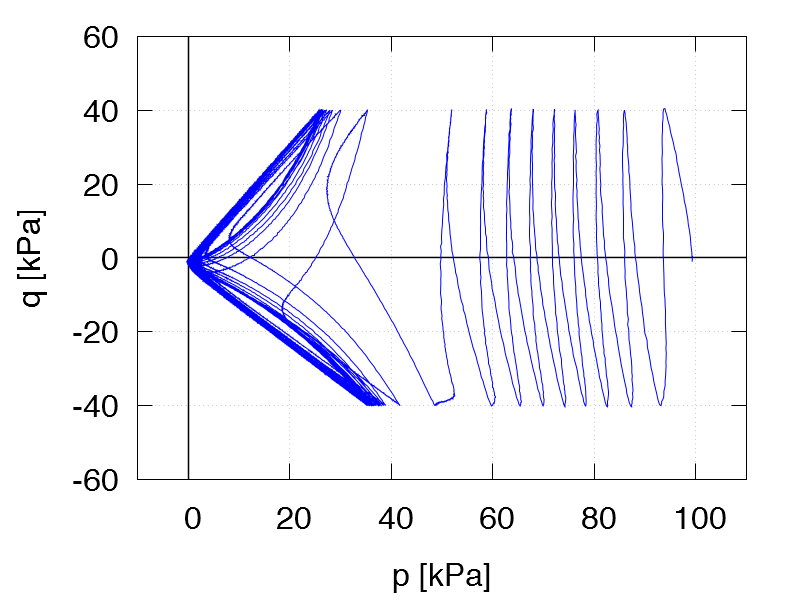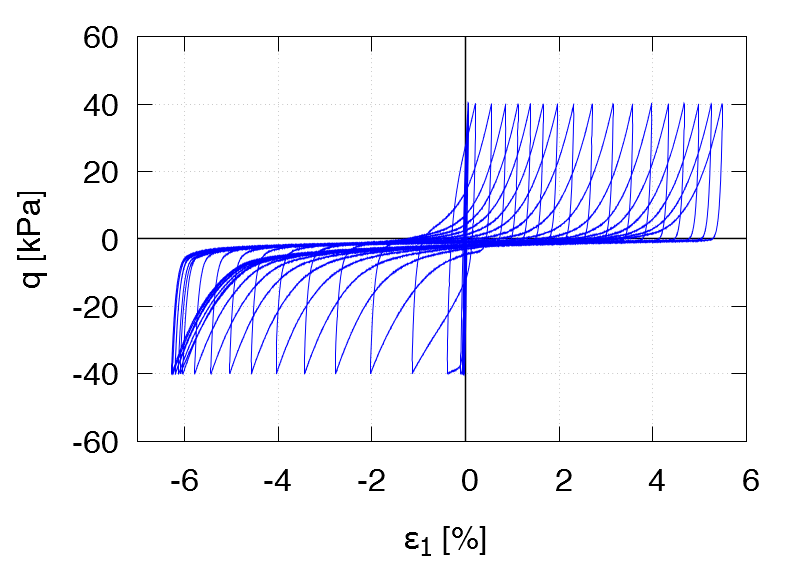TCUI20 ID0 = 0.79 p0 = 100 kPa qampl = 50 kPa 1st drained cycle Test data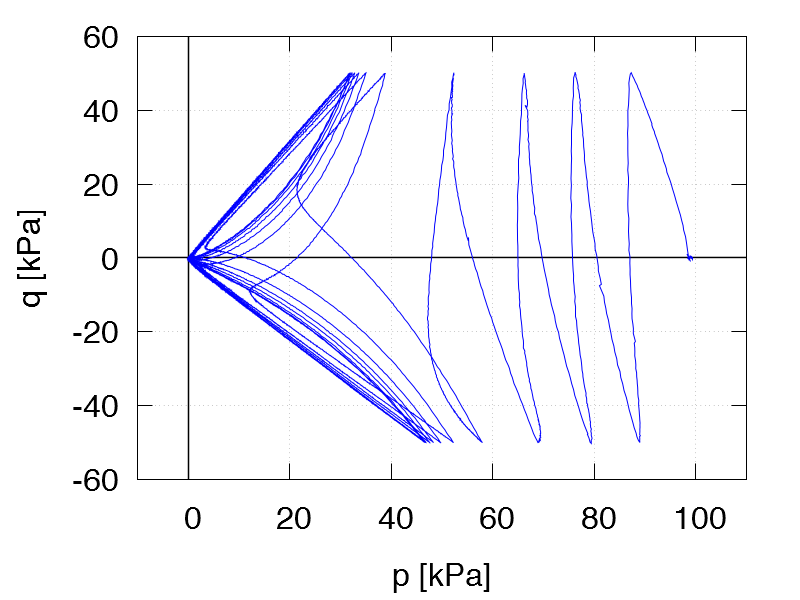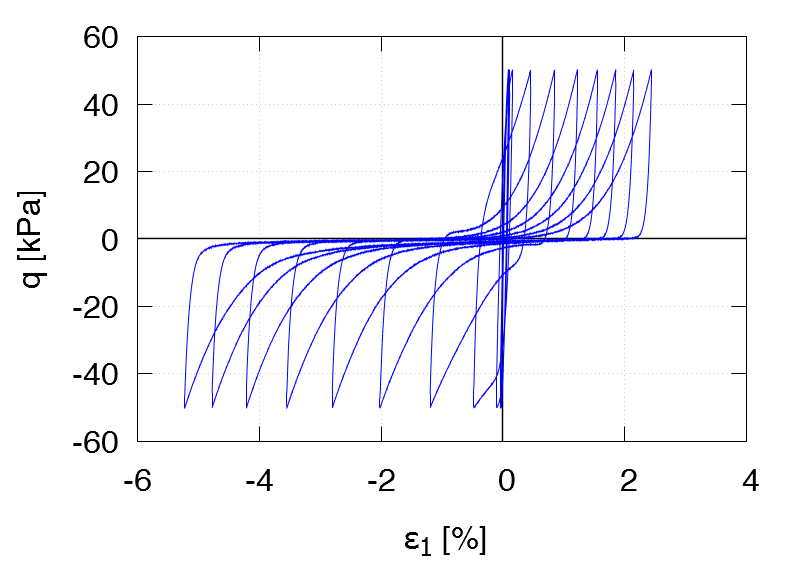TCUI21 ID0 = 0.80 p0 = 200 kPa qampl = 60 kPa 1st drained cycle Test data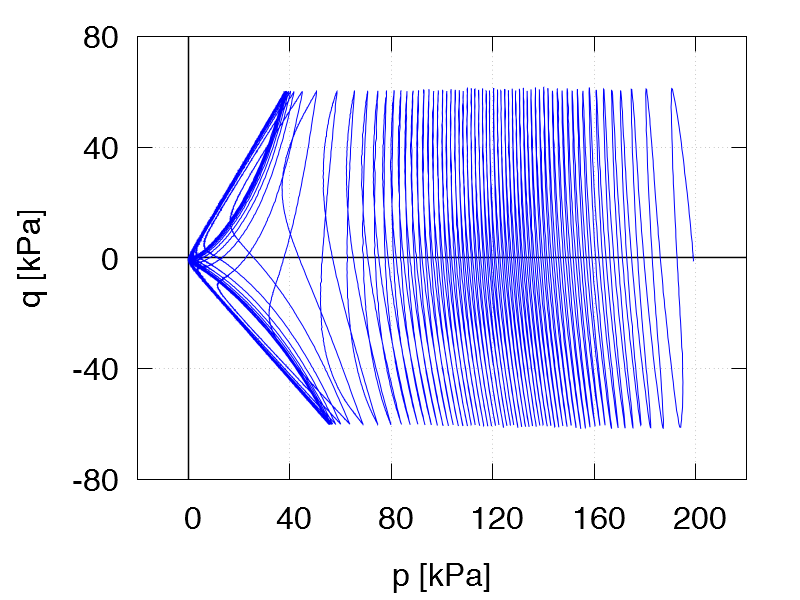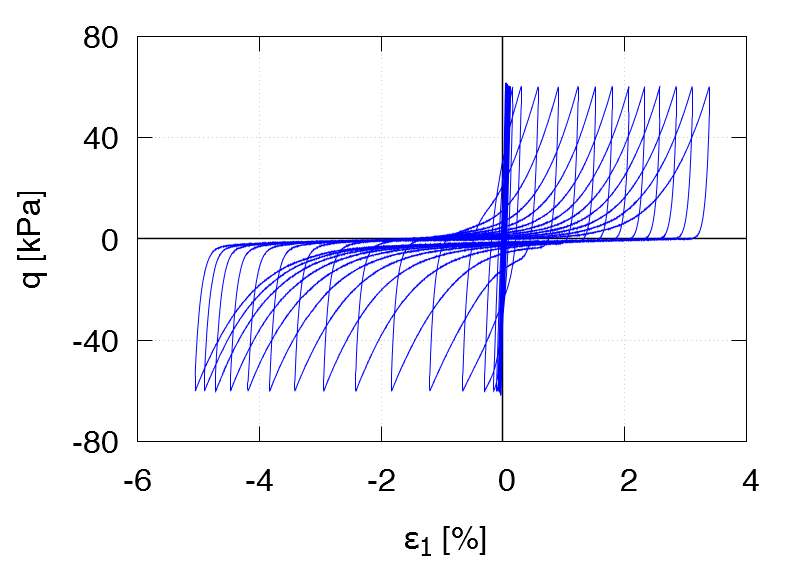TCUI22 ID0 = 0.82 p0 = 300 kPa qampl = 90 kPa 1st drained cycle Test data